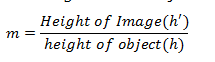﻿ Sign Convention(Spherical Lenses), Lens Formula and Magnification# Refraction of Light Class 10

## Sign Convention for Spherical Lenses

1. All the distances are measured from the optical center of the lens.
2. The distances measured in the same direction as that of incident light are taken as positive.
3. The distances measured against the direction of incident light are taken as negative.
4. The distances measured upward and perpendicular to the principle axis are taken as positive.
5. The distances measured downwards and perpendicular to principle axis is taken as negative.

## Lens Formula and Magnification

• Lens Formula gives the relationship between object distance (u), image image-distance (v) and the focal length (f ) and is expressed as
$\frac{1}{f} = \frac{1}{v} - \frac{1}{u}$
This formula is valid in all situations for any spherical lens.
• The magnification produced by a lens is defined as the ratio of the height of the image and the height of the object.• Magnification produced by a lens is also related to the object-distance u, and the image-distance v and is given by
$m = \frac{v}{u}$
• The power of a lens is defined as the reciprocal of its focal length. It is represented by the letter P. The power P of a lens of focal length f is given by
$P = \frac{1}{f}$
• Power of a convex lens is positive and that of a concave lens is negative.
• The SI unit of power of a lens is ‘dioptre’. It is denoted by the letter D.
• 1 dioptre is the power of a lens whose focal length is 1 meter so, 1D=1m–1.

Given below are the links of some of the reference books for class 10 Science.

You can use above books for extra knowledge and practicing different questions.

### Practice Question

Question 1 Which among the following is not a base?
A) NaOH
B) $NH_4OH$
C) $C_2H_5OH$
D) KOH
Question 2 What is the minimum resistance which can be made using five resistors each of 1/2 Ohm?
A) 1/10 Ohm
B) 1/25 ohm
C) 10 ohm
D) 2 ohm
Question 3 Which of the following statement is incorrect? ?
A) For every hormone there is a gene
B) For production of every enzyme there is a gene
C) For every molecule of fat there is a gene
D) For every protein there is a gene

Note to our visitors :-

Thanks for visiting our website.